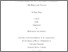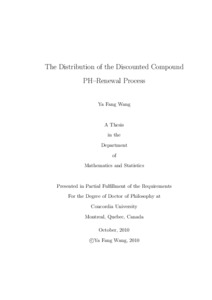Title:

# The Distribution of the Discounted Compound PH-Renewal Process

Wang, Ya Fang (2010) The Distribution of the Discounted Compound PH-Renewal Process. PhD thesis, Concordia University.Preview
Text (application/pdf)
Wang_PhD_S2011.pdf - Accepted Version
1MB

## Abstract

The family of phase–type (PH) distributions has many useful properties such as closure under convolution and mixtures, as well as rational Laplace transforms. PH distributions are
widely used in applications of stochastic models such as in queueing systems, biostatistics and engineering. They are also applied to insurance risk, such as in ruin theory.

In this thesis, we extend the work of Wang (2007), that discussed the moment generating function (mgf) of discounted compound sums with PH inter–arrival times under a net interest δ ̸= 0. Here we focus on the distribution of the discounted compound sums. This represents a generalization of the classical risk model for which δ = 0.

A differential equation system is derived for the mgf of a discounted compound sum with PH inter–arrival times and any claim severity if its mgf exists. For some PH inter–arrival times, we can further simplify this differential equation system. If inter–arrival times have a PH distribution of order 2, then second–order homogeneous differential equations are developed. By inverting the corresponding Laplace transforms, the extended density functions and cumulative distribution functions are also obtained. In addition, the series and transformation methods for solving differential equations is proposed, when the mean of inter–arrival times is small.

Applications such as stop–loss premiums, and risk measures such as VaR and CTE are investigated. These are compared for different inter–arrival times. Some numerical examples
are given to illustrate the results.

Finally, asymptotical results are discussed, when the mean inter–arrival time goes to zero. For a fixed time, the asymptotic normal distribution is derived for discounted compound renewal sums.

Divisions: Concordia University > Faculty of Arts and Science > Mathematics and Statistics Thesis (PhD) Wang, Ya Fang Concordia University ﻿Ph. D. Mathematics 1 December 2010 Garrido, José and Léveillé, Ghislain 6973 YA FANG WANG 13 Jun 2011 13:58 18 Jan 2018 17:29
All items in Spectrum are protected by copyright, with all rights reserved. The use of items is governed by Spectrum's terms of access.

Repository Staff Only: item control pageResearch related to the current document (at the CORE website)
Back to top